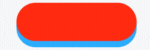## What is FAR?

Floor area ratio (FAR) is the ratio of a building`s total floor area to the size of the plot of land upon which it is built### What is The Difference Between FAR & FSI​

Floor Area Ratio (FAR) or Floor Space Index (FSI) denote the maximum floor space that can be constructed on a piece of land. Both FAR & FSI are the same .

Maximum Area you can build

Maximum number of Floors you can build

### FAR In Faridabad (As per By Laws )

Area of Plot Gurgaon
(Sq mtrs)
Max Ground Coverage (%)Real FAR
(Floor Area Ratio)
Purchasable FARTotal FAR
Below 756616599264
Above 75 -1006616599264
Above 100 – 15066145119264
Above 150 – 20066145119264
Above 200 – 25066145119264
Above 250 – 35060125115240
Above 350 – 50060120120240
Above 50060100140240

#### Click on the link to Visit the government site: FAR Faridabad

Example:

Assume that a plot of land comes with a floor area ratio (FAR) of 3.0, and the plot area is 1,000 square feet.

FAR = 1000 Sq. Feet plot area x 3.0 = 3000 square feet.

What is the formula for number of Floors as per Maximum Ground coverage & FAR

Total Plot area x Maximum Ground Coverage (%) = A

Total Plot area x Permissible FAR = B

Number of floors permissible = B ÷ A

Let us understand why the floor area ratio (FAR) is important and has been defined by the Government: Any land plot or an area in the city has a limited capacity of taking building load and fundamentally it is not right to put additional stress beyond this capacity. This is true for both your plot as well as the whole city.
Hence the FAR has been defined for the well being of all the Citizens in order to keep them safe. FAR is also thus known as Safe-Load Factor
The FAR guidelines are defined by the Municipalities and different localities and areas can have different FAR based on the safety and load bearing capacity of that area The FAR Ratio will differ depending on the population density, construction-related activities, growth patterns, and the nature of the building’s space or land.

## What is not included in FAR

Unoccupied areas like parking, garages, elevator shafts, and basements are not included in the calculation of a floor area ratio*.

For more details when you speak with our Sales Representatives and the Architect, we will be able to give you the exact calculation for your plot as well as the areas not included in floor area ratio (FAR) your locality